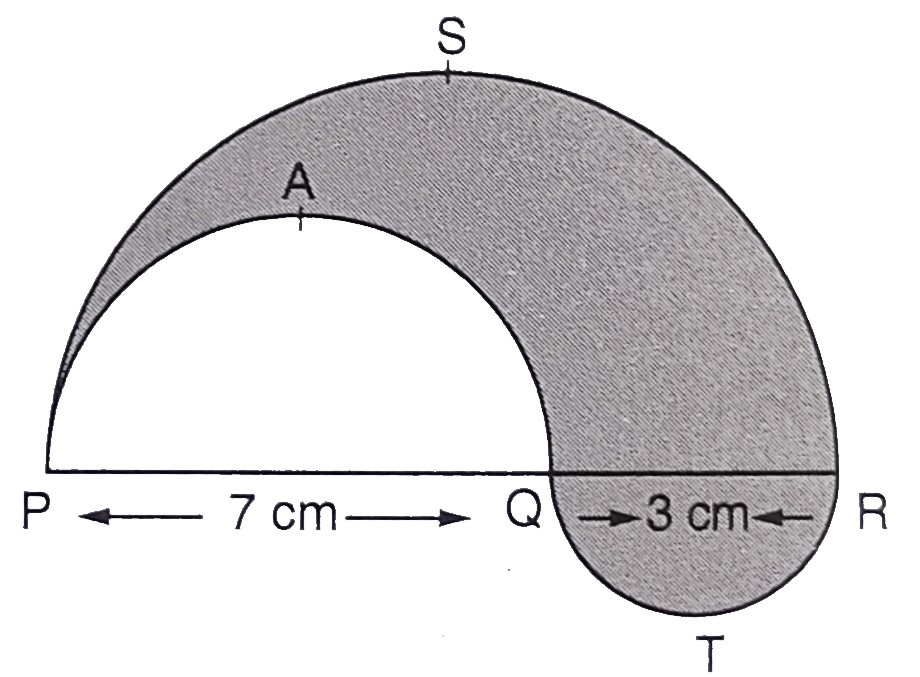"
">

# In the below figure, PSR, RTQ and $P A Q$ are three semi-circles of diameters $10 \mathrm{~cm}, 3 \mathrm{~cm}$ and $7 \mathrm{~cm}$ respectively. Find the perimeter of the shaded region."

Given:

PSR, RTQ and $P A Q$ are three semi-circles of diameters $10 \mathrm{~cm}, 3 \mathrm{~cm}$ and $7 \mathrm{~cm}$ respectively.

To do:

We have to find the perimeter of the shaded region.

Solution:

Radius of semicircle $PSR=r_1 =\frac{10}{2}= 5\ cm$
Radius of semicircle $PAQ= r_2 =\frac{7}{2}\ cm$
Radius of semicircle $QTR=r_3 =\frac{3}{2}\ cm$

Therefore,

Perimeter of the shaded region $=\pi r_{1}+\pi r_{2}+\pi r_{3}$

$=\pi(5+\frac{7}{2}+\frac{3}{2})$

$=\pi \times 10$

$=\frac{22}{7} \times 10$

$=\frac{220}{7}$

$=31.41 \mathrm{~cm}$

The perimeter of the shaded region is $31.41\ cm$.

Updated on: 10-Oct-2022

68 Views